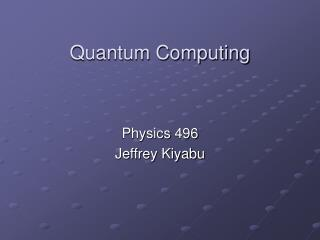DownloadDownload PresentationQuantum Computing

# Quantum Computing

Télécharger la présentation## Quantum Computing

- - - - - - - - - - - - - - - - - - - - - - - - - - - E N D - - - - - - - - - - - - - - - - - - - - - - - - - - -
##### Presentation Transcript

1. Quantum Computing Physics 496 Jeffrey Kiyabu

2. Quantum Computing • Bits & Qubits • Quantum phenomena A. Superposition B. Parallelism C. Interference • Computation A. Unitary operators (logic gates) B. Algorithms • Technological/Systematic Obstacles A. Decoherence B. Hardware architecture

3. Bits & Qubits Bit – 0 or 1 Qubit – quantum mechanical two-state system (spin up, spin down or 0, 1); a system of n qubits represents 2n possible states

4. Quantum Phenomena • Superposition • Parallelism • Interference

5. Computation • Unitary operators (logic gates) e.g. not-gate • Algorithm – sequence of logic gates operating on a system e.g. quantum search algorithm

6. Obstacles • Decoherence – information in the system lost to environment • Hardware architecture

7. Quantum Computing • Bits & Qubits • Quantum phenomena A. Superposition B. Interference C. Parallelism • Computation A. Unitary operators (logic gates) B. Algorithms • Technological/Systematic Obstacles A. Decoherence B. Hardware architecture

8. “Where a calculator on the Eniac is equipped with 18000 vacuum tubes and weighs 30 tons, computers in the future may have only 1000 tubes and weigh only 1 1/2 tons," -Popular Mechanics, March 1949.

9. References 1. Ekert, Arthur., et al. “Basic concepts in quantum computation.” Quantiki. (2006): 16 April 2007. <http://www.quantiki.org/wiki/index.php/Basic_concepts_in_quantum_ computation>. 2. Grover, Lov. Quantum Computing. “The Sciences.” August 1999. <http://cryptome.org/qc-grover.htm>. 3. Hirvensalo, Mika. Quantum Computing. 2nd edition. Ed. Rozenberg, G. Berlin: Springer-Verlag, 2004. 4. Mathematics of Quantum Computation. Ed. Brylinski, Ranee K. and Chen, Goong. Boca Raton: Chapman & Hall/CRC, 2002. 5. West, James. “The Quantum Computer.” An introduction to Quantum Computing. 28 April 2000. <http://www.cs.caltech.edu/~westside/quantum-intro.html >. 6. “Quantum computer.” Wikipedia. 16 April 2007. <http://en.wikipedia.org/wiki/Quantum_computer>.

10. questions